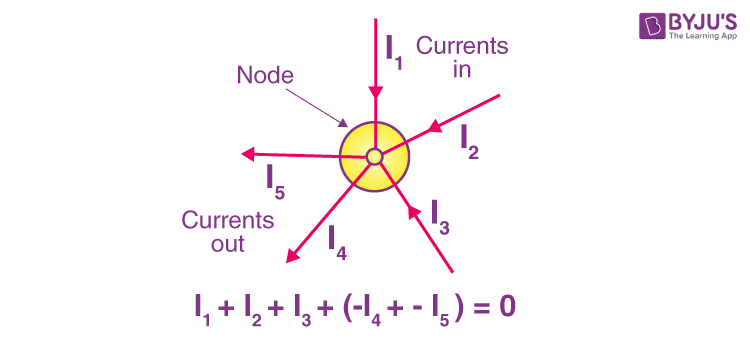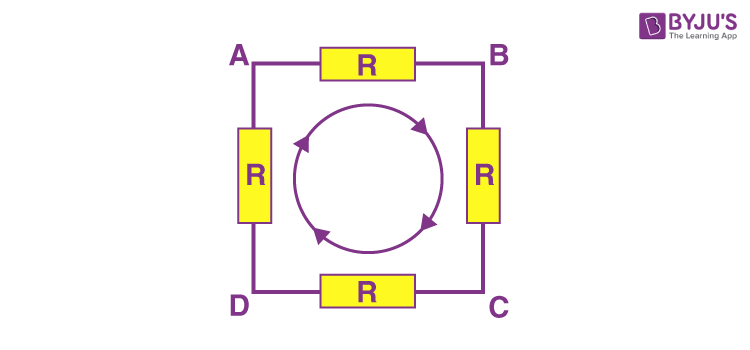# State and explain Kirchhoff's Law

Kirchhoff’s laws are one of the fundamental laws that find applications in electrical engineering for formulating circuits. There are two laws that makeup Kirchhoff’s law, and they are:

## Kirchhoff’s Current Law (KCL)

KCL is also known as Kirchhoff’s first law or junction rule. The principle of this law is to conserve the electric charge. The law states that the amount of current flowing into a node is equal to the sum of currents flowing out of it. For performing the nodal analysis in Ohm’s law, KCL is used.The currents I1, I2, and I3 entering the node are considered positive values, while the currents I4 and I5 leaving the nodes are considered negative values. This can be expressed mathematically as follows:

I1 + I2 + I3 – I4 – I5 = 0

## Kirchhoff’s Voltage Law (KVL)

KVL is also known as Kirchhoff’s second law or loop law. The principle of this law is to conserve energy. The law states that the sum of voltages is in a closed-loop is zero. The total amount of energy gained is equal to the energy lost per unit charge.VAB + VBC + VCD + VDA = 0

When you start at any point in the loop and keep going in the same direction, you’ll notice that the voltage drops in all directions, whether negative or positive, and you’ll come back to the same point. It is important to maintain either a counterclockwise or a clockwise rotation; otherwise, the final voltage value will not be zero.

4 (31)

Upvote (37)

#### Choose An Option That Best Describes Your Problem

Thank you. Your Feedback will Help us Serve you better.

#### 1 Comment

1. stephano

i love this site is quite interesting